Chapter 4 Class 10 Quadratic Equations

Class 10
Important Questions for Exam - Class 10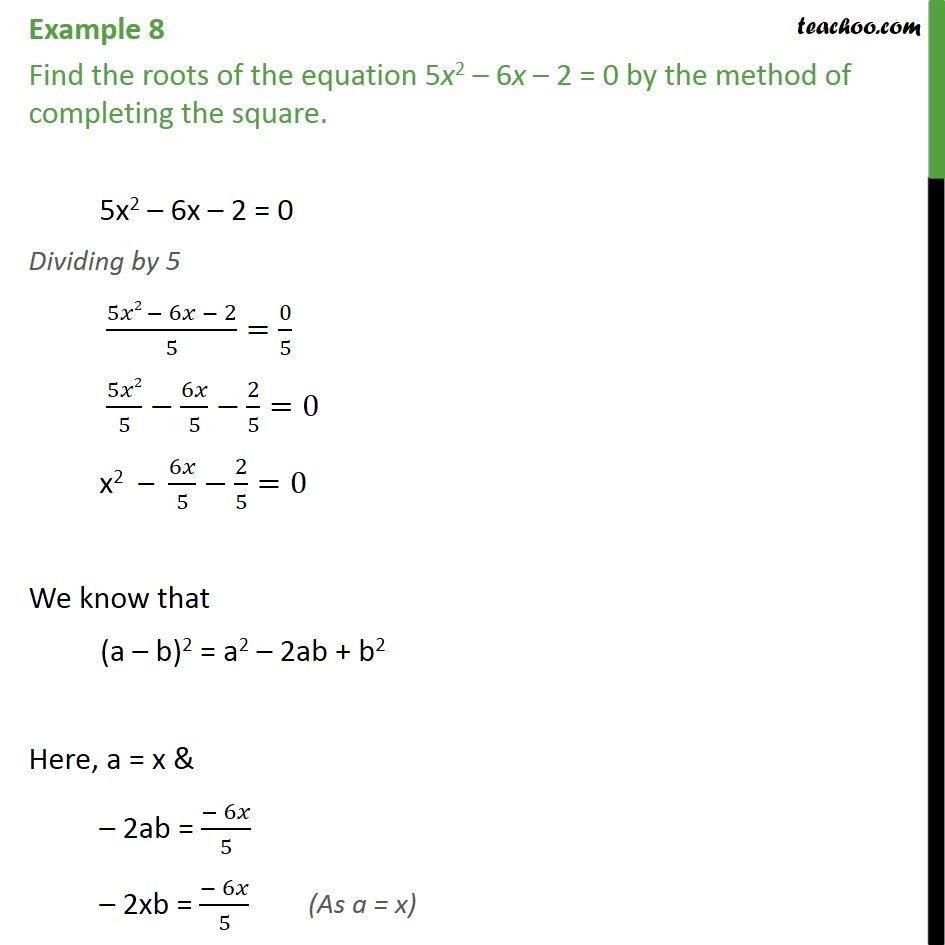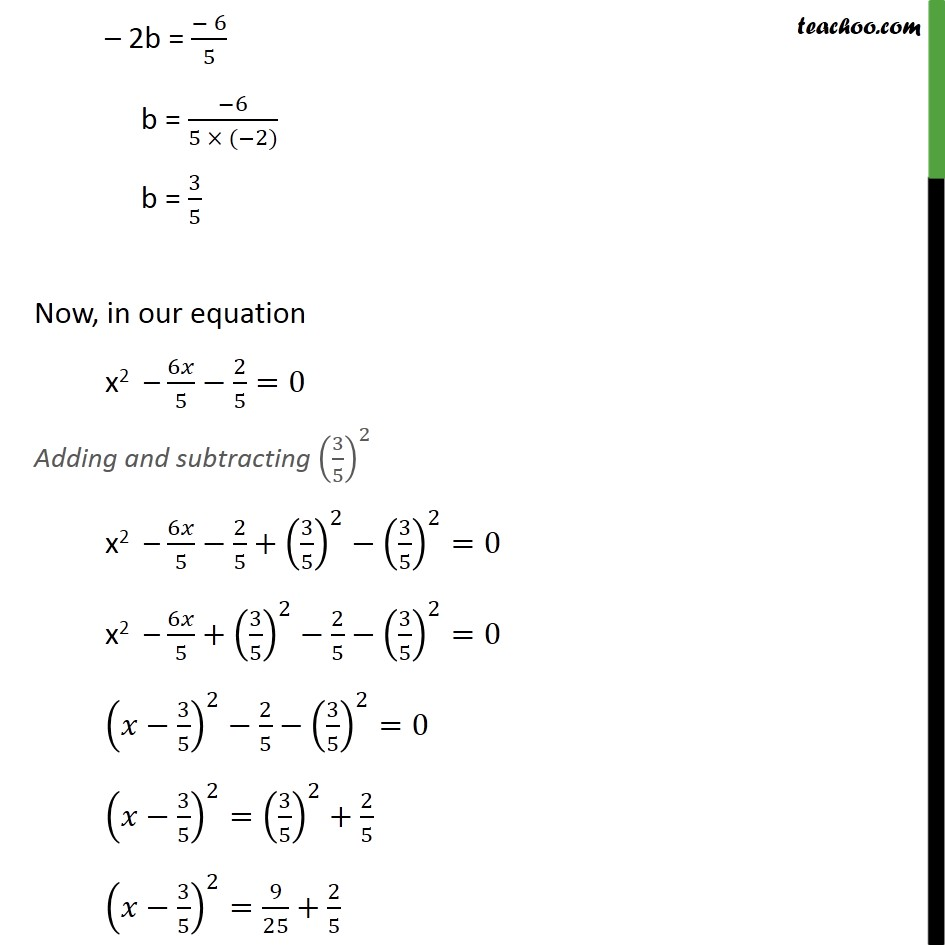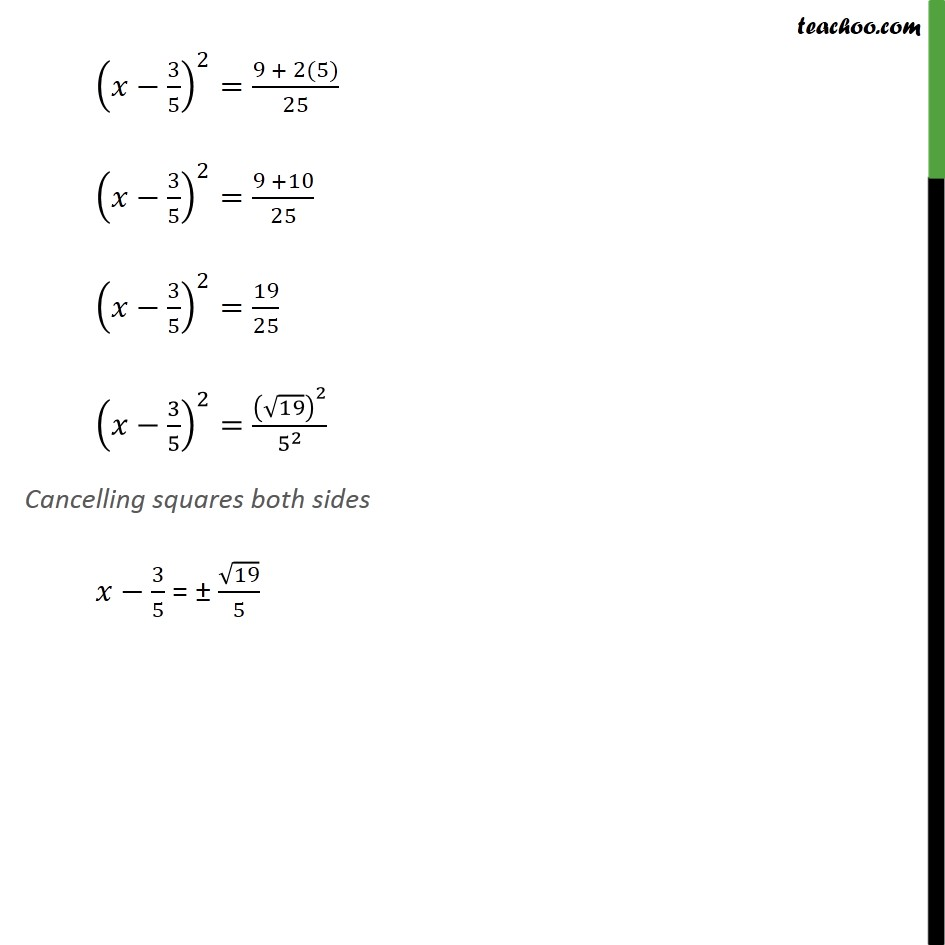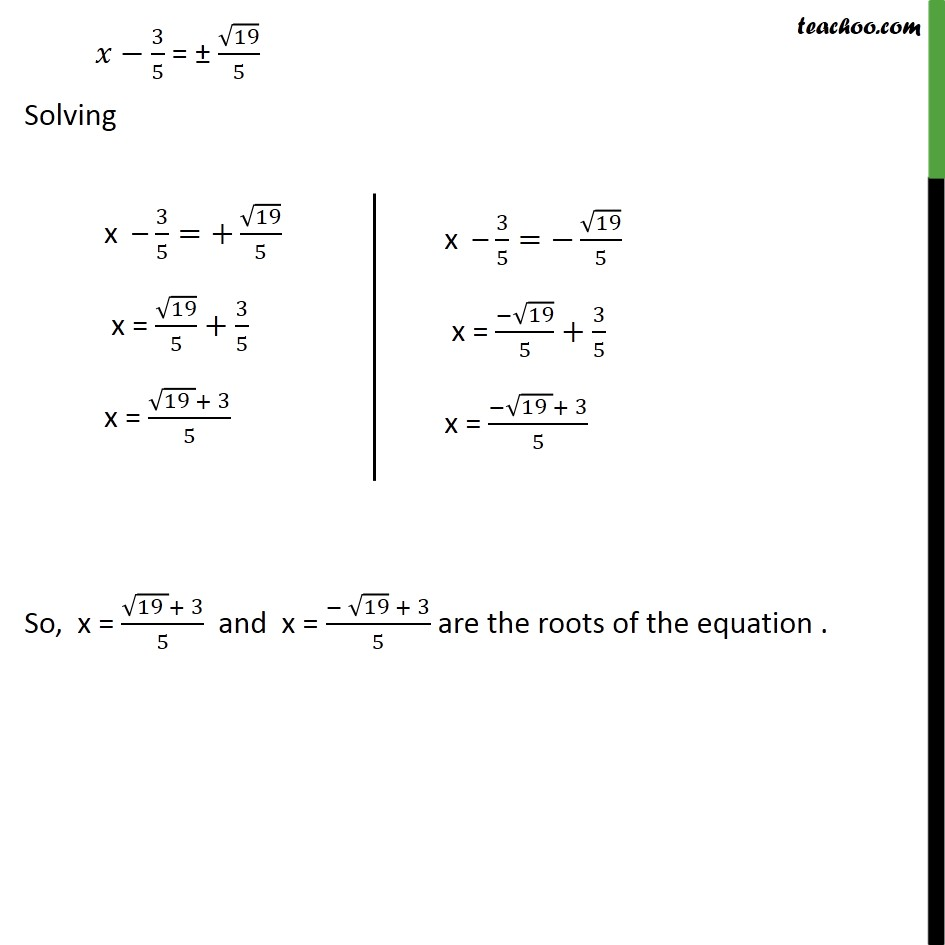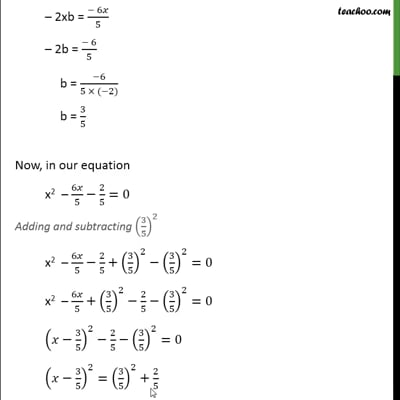This video is only available for Teachoo black users

Introducing your new favourite teacher - Teachoo Black, at only ₹83 per month

### Transcript

Example 8 Find the roots of the equation 5x2 6x 2 = 0 by the method of completing the square. 5x2 6x 2 = 0 Dividing by 5 (5 2 6 2)/5=0/5 5 2/5 6 /5 2/5=0 x2 6 /5 2/5=0 We know that (a b)2 = a2 2ab + b2 Here, a = x & 2ab = ( 6 )/5 2xb = ( 6 )/5 2b = ( 6)/5 b = ( 6)/(5 ( 2)) b = 3/5 Now, in our equation x2 6 /5 2/5=0 Adding and subtracting (3/5)^2 x2 6 /5 2/5+(3/5)^2 (3/5)^2=0 x2 6 /5+(3/5)^2 2/5 (3/5)^2=0 ( 3/5)^2 2/5 (3/5)^2=0 ( 3/5)^2=(3/5)^2+2/5 ( 3/5)^2=9/25+2/5 ( 3/5)^2=(9 + 2(5))/25 ( 3/5)^2=(9 +10)/25 ( 3/5)^2=19/25 ( 3/5)^2=( 19)^2/5^2 Cancelling squares both sides 3/5 = 19/5 3/5 = 19/5 Solving So, x = ( (19 )+ 3)/5 and x = ( 19 + 3)/5 are the roots of the equation .